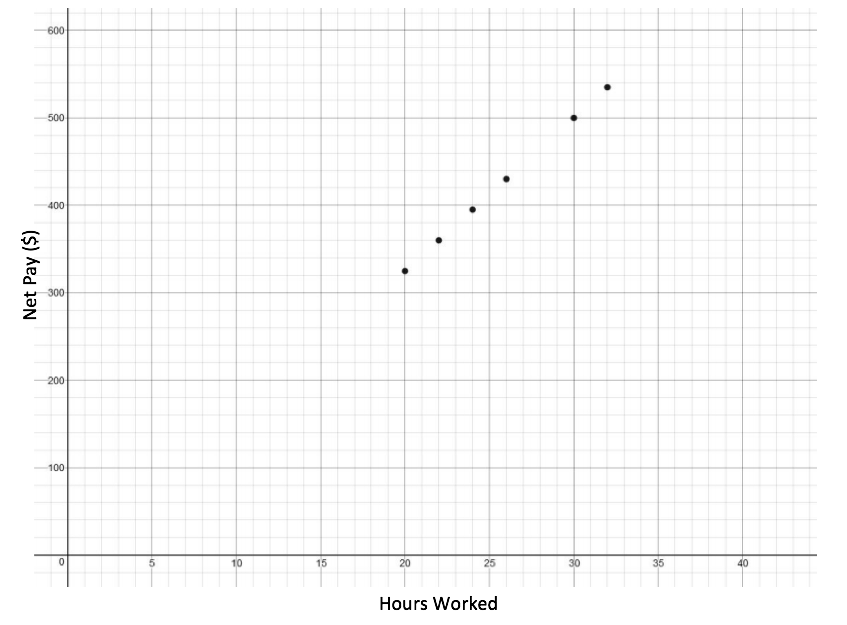## Putting It Together: Calculations and Solving Equations

Kareem works as a checkout clerk at Best Food Supermarket and received the following report for his pay over the past couple months:

 Employee Name Kareem Martin Employee ID #32156 Pay Period Ending Hours Net Pay Dec 11, 2016 20 $325.00 Dec 4, 2016 26$430.00 Nov 27, 2016 32 $535.00 Nov 20, 2016 22$360.00 Nov 13, 2016 24 $395.00 Nov 6, 2016 30$500.00

He wants to make sure he got paid correctly, so he’s going to analyze the information he has been sent. Kareem decides it will be easier if he can visualize the data, so he creates six ordered pairs from the table that he can graph:

$(20,325), (26,430), (32,535), (22,360), (24,395), (30,500)$

He then plots those points on a graph:Kareem can observe a few things from this graph:

1. He can determine a trend in his pay
2. He can calculate the rate of change to find his hourly rate
3. He can analyze the values to see if he’s being paid correctly

Let’s solve those problems!

1. Using a straight edge he can see that the data rises to the right in what looks to be a perfectly straight line.
2.  We’ll call $\left(20,325\right)$ point #1 and $\left(30,500\right)$ point #2. $\stackrel{{x}_{1},{y}_{1}}{\left(20,325\right)}\text{and}\stackrel{{x}_{2},{y}_{2}}{\left(30,500\right)}$ Use the slope formula to find the rate of change. $m={\Large\frac{{y}_{2}-{y}_{1}}{{x}_{2}-{x}_{1}}}$ Substitute the values in the slope formula: $y$ of the second point minus $y$ of the first point $m={\Large\frac{500 - 325}{{x}_{2}-{x}_{1}}}$ $x$ of the second point minus $x$ of the first point $m={\Large\frac{500 - 325}{30 - 20}}$ Simplify the numerator and the denominator. $m={\Large\frac{175}{10}}$ $m=17.5$
3. If Kareem makes $17.5$/hr, then shouldn’t he be paid $350$ for $20$ hours of work? And for $30$ hours of work he’s paid $25$ less than his hourly wage too. I looks like $25$ is being taken off each of his paychecks.

Can you determine an equation to represent his pay based on the number of hours he worked?

Kareem is getter paid $17.5$/hr, since that is the rate of change between his paychecks of different hour amounts. However each amount in the net pay column is $25$ less than the number of hours multiplied by his hourly wage. Kareem then remembers that he has $25$ deducted and put into his Health Savings Account each paycheck. So he makes $17.5$ per hour worked minus $25$. If we translate into an equation, Kareem’s pay equals:

$P=17.5h-25$

How much money would Kareem make if he works 28 hours?

Now that we have an equation to represent Kareem’s pay, we can plug in $28$ for $h$

$P=17.5h-25$

$P=17.5(28)-25$

$P=17.5(28)-25$

$P=458$

We could also have drawn a line through all the points on the graph, found $28$ on the $x$-axis, and and figured out what the $y$ value would be when we hit the line. With these larger numbers that likely wouldn’t have been as accurate as using the equation.

## Contribute!

Did you have an idea for improving this content? We’d love your input.